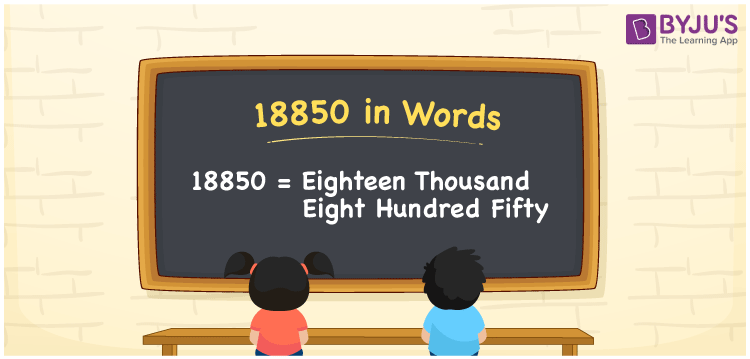# 18850 in Words

We can write the number 18850 in words as Eighteen thousand eight hundred fifty. If you purchased a camera that costs Rs. 18850, you can say, “I purchased a camera worth Eighteen thousand eight hundred fifty rupees”. That means we can express the number 18850 in words as Eighteen thousand eight hundred fifty. In this article, you can understand how to convert the number 18850 into words with the help of a place value chart.

 18850 in words Eighteen thousand eight hundred fifty Eighteen thousand eight hundred fifty in Numbers 18850

## 18850 in English Words

Generally, we use the English alphabet to write numbers in words. Thus, we can spell 18850 in English words as “Eighteen thousand eight hundred fifty”.## How to Write 18850 in Words?

As we can see, the number 18850 has five digits. So, to convert the number 18850 into words, we need to make a place value chart with five columns as shown below.

 Ten thousands Thousands Hundreds Tens Ones 1 8 8 5 0

Here, ones = 0, tens = 5, hundreds = 8, thousands = 8, ten thousands = 1

These digits can be expanded as follows.

1 × Ten thousand + 8 × Thousand + 8 × Hundred + 5 × Ten + 0 × One

= 1 × 10000 + 8 × 1000 + 8 × 100 + 5 × 10 + 0 × 1

= 10000 + 8000 + 800 + 50

= Ten Thousand + Eight thousand + Eight hundred + Fifty

= Eighteen thousand + Eight hundred fifty {since ten + three = thirteen}

= Eighteen thousand eight hundred fifty

18850 is a natural number that is the successor of 18849 and predecessor of 18851.

18850 in words – Eighteen thousand eight hundred fifty

Is 18850 an odd number? – No

Is 18850 an even number? – Yes

Is 18850 a prime number? – No

Is 18850 a composite number? – Yes

Is 18850 a perfect square number? – No

Is 18850 a perfect cube number? – No

## Frequently Asked Questions on 18850 in Words

### What is the word form of 18850?

The word form of the number 18850 is Eighteen thousand eight hundred fifty.

### How to write Rs. 18850 in words on a cheque?

On a cheque, we can write Rs. 18850 in words as “Eighteen thousand eight hundred fifty rupees only”.

### Write the value of 18850 – 16965 in words.

18850 – 16965 = 1885 Thus, the value of 18850 – 16965, i.e., 1885 in words is One thousand eight hundred eighty-five.